# Choosing The Right Year 11 Mathematics Course

In this post, we answer some frequently asked questions from Year 10 students about choosing the right Mathematics course for Year 11:

• When does the new Year 11 & 12 (Stage 6) Mathematics Syllabus start?
• What are the differences between Mathematics Advanced vs Mathematics Extension 1?
• Should I choose Mathematics Advanced or Mathematics Extension 1?
• How important are Year 11 Mathematics courses in the HSC?
• How do the different Mathematics courses scale?

## The current Year 11 Mathematics syllabus

Year 11 Mathematics is the first year of Stage 6 Mathematics.

• Year 9 and 10 Mathematics is known as Stage 5 Mathematics
• Year 11 and 12 Mathematics is known as Stage 6 Mathematics

For Year 11 students, there are three different courses of study. Below are the NESA links to the course outlines:

• Mathematics Standard and Standard 2
• Mathematics Extension 1

For Year 12 students, there are four different courses of study:

• Mathematics Standard and Standard 2
• Mathematics Extension 1
• Mathematics Extension 2

## Choosing the right Year 11 Mathematics course (Maths Standard, Maths Advanced, Maths Extension 1)

The different levels of Mathematics have different requirements for student competency and ability. To help students determine which Mathematics course is appropriate for them, we have created a flowchart that illustrates the level of achievement students need to attain for the different levels of Mathematics courses.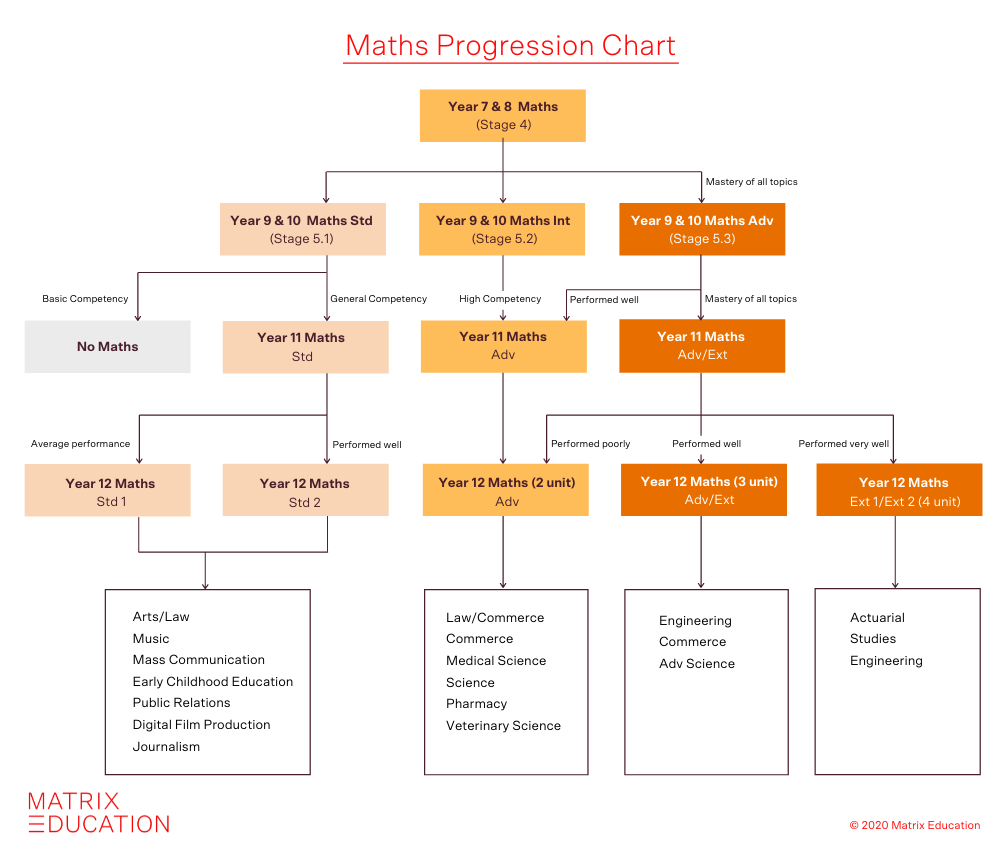The Year 11 and 12 Maths Standard 1 and 2 courses are aimed at giving students proficiency in practical aspects of Maths for entry into the workforce. Students’ performance in Year 10 Mathematics should determine the level of Year 11 Mathematics they choose to study.

• Year 11 and 12 Maths Standard and Standard 2 is aimed at further developing students Maths skills, but not to the level required for university degrees requiring Maths Advanced. Students who do well at this and wish to study a course at university requiring Maths Prerequisites will need to undertake a bridging course, although this subject will help prepare for such bridging study.
• The Year 11 and 12 Mathematics Advanced course is intended for students who have demonstrated general competency in all the skills in the Year 10 Mathematics Advanced Course. If students struggled in Year 10 Mathematics Advanced, they should consider taking the Year 11 Mathematics Advanced course.
• If students performed well in Year 10 Mathematics Advanced, they should consider taking the Year 11 Mathematics Extension 1 course.
• The Year 11 and 12 Mathematics Extension 1 course is intended for students who have demonstrated a mastery of the skills in the Year 10 Mathematics Advanced course. If students performed well in Year 10 Mathematics Advanced, they should consider taking the Year 11 Mathematics Extension 1 course.

Various university courses now require Mathematics Advanced or higher as prerequisites

Universities are starting to introduce Mathematics prerequisites or/and recommended subjects with minimum HSC result for various courses.

Click here for a table of the University of Sydney degrees that will require a Maths prerequisite.

NESA recommends:

• The Mathematics Advanced (2 Unit) course as the most appropriate for university courses such as in the Life Sciences, Business, Finance, Technology, and Education.
• The Mathematics Extension 1 course as the most appropriate for university courses such as Physics, Chemistry, Engineering, Statistics, and Computer Science.

## Why is Mathematics Extension 1 referred to as 3 Unit Mathematics?

The content of the Mathematics Extension 1 course includes the whole of the Mathematics Advanced (2 unit) course. Therefore, students sit HSC exams for:

• The Year 12 Mathematics Advanced and
• The Year 12 Mathematics Extension 1.

For this reason, the Year 12 Mathematics Advanced course is assigned 2 units of HSC marks and the Year 12 Mathematics Extension 1 course is assigned 1 unit of HSC marks. Hence the total number of units for this course is 3 units.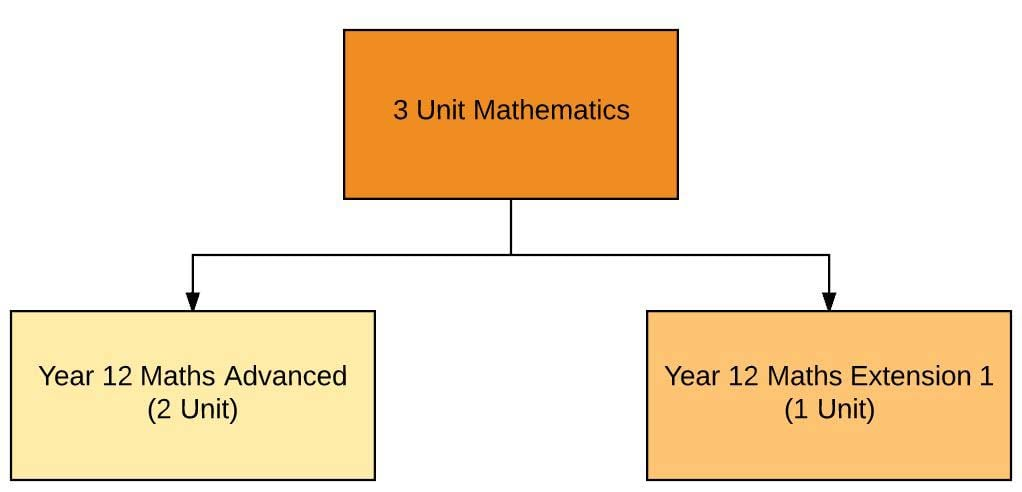## Comparing Mathematics courses: Mathematics Standard 2 Vs Advanced vs Mathematics Extension 1

The Year 11 Mathematics Advanced and Extension 1 topics are listed below. Please note that in Year 11 Maths Extension 1, students will learn both Year 11 Maths Advanced and Extension 1 topics.

 Table: Year 11 Mathematics Advanced and Extension 1 Syllabus Year 11 Maths Standard Year 11 Maths Advanced Year 11 Maths Extension  1 MS-A1: Formulae and Equations Basic arithmetic and algebra (1.1 – 1.4) Other inequalities (1.4E) MS-A2: Linear Relationships Real functions (4.1 – 4.4) Circle geometry (2.6 – 2.10) MS-F1 (F1.1): Interest and depreciation Trigonometric ratios (5.1 – 5.5) Further trigonometry (5.6 – 5.9) MS-M1 Applications of Measurement (M1.1) Linear functions (6.1-6.5, 6.7) Angles between two lines (6.6) MS-F1 Money Matters The quadratic polynomial and the parabola (9.1 – 9.5) Internal and external division of lines into given ratios (6.7E) MS-M2 Working with Time Plane geometry (2.1 – 2.4) Parametric representation (9.6) MS-S1 Data Analysis Tangent to a curve and derivative of a function (8.1 – 8.9) Permutations and combinations (18.1) MS-S2 Relative Frequency and Probability Polynomials (16.1 – 16.3) Harder applications of the Preliminary 2 Unit course

Depending on schools, the Year 11 Mathematics Extension 1 program will vary.

• Some schools choose to complete Year 11 Mathematics Advanced topics first before teaching Year 11 Mathematics Extension 1 topics.
• Most schools choose to teach Year 11 Mathematics Advanced and Extension 1 topics one after the other.

At Matrix, we teach Year 11 Mathematics and Extension 1 topics one after the other. The Matrix Year 11 Mathematics Standard 2, Advanced, and Extension 1 course programs are shown below:

Table: Matrix Year 11 Mathematics Program
Period Year 11 Maths Standard Year 11 Maths Advanced Year 11 Maths Extension 1
##### Launching September 2021
Basic Arithmetic and Algebra
Absolute Values
Linear Functions
Linear Functions
Non-Linear Curves
Absolute Values and Inequalities
##### Launching September 2021
Functions and Relations
Trigonometric Ratios
Trigonometric ratios
Locus
Limits of the Derivative
##### Launching September 2021
Plane geometry
Locus and Parabola
Polynomials
Geometrical Applications of Differentiation
Parametric Representation
##### Launching September 2021
Introductory Calculus
Probability
Revision of Preliminary Topics
Sequences and Series
Circle Geometry
Integration
Volumes

The Year 12 Mathematics Advanced, Standard 2, and Extension 1 topics are listed below. Please note that Year 12 Maths Extension 1 students will learn both Year 12 Maths Advanced and Extension 1 topics.

 Table: Year 12 Mathematics Standard 2,  Advanced, and Extension 1 Syllabus Year 12 Maths Standard 2 Year 12 Maths Advanced Year 12 Maths Extension 1 MS-A4 Types of Relationships Coordinate methods in geometry (6.8) Method of integration (11.5) MS-F4 Investments and Loans Applications of geometrical properties (2.5) Primitive of sin(2x) and cos(2x) (13.6E) MS-N2 Network Concepts Geometrical applications of differentiation (10.1-10.8) Exponential growth and decay equation (14.2E) MS-F5 Annuities Integration (11.1 – 11.4) Velocity and acceleration as a function of ‘x’ (14.3E) MS-M6 Non-right-angled trigonometry Trigonometric functions (including applications of trigonometric ratios) (13.1 – 13.6, 13.7) Projectile motion (14.3E) MS-M7 Rates and Ratios Logarithmic and exponential functions (12.1 – 12.5) Simple harmonic motion (14.4) MS-N3 Critical Path Analysis Applications of calculus to the physical world (14.1 – 14.3) Inverse functions and inverse trigonometric functions (15.1 – 15.5) MS-S4 Bivariate Data Analysis Probability (3.1 – 3.3) Induction (7.4) MS-S5 The Normal Distribution Series (7.1 – 7.3) and Series applications (7.5) Binomial theorem (17.1 – 17.3) Further probability (18.2) Iterative methods for estimating roots (16.4) Harder applications of HSC 2 Unit topics

At Matrix, we teach Year 12 Mathematics and Extension 1 topics one after the other. The Matrix Year 11 Mathematics Standard 2, Advanced, and Extension 1 course programs are shown below:

Table: Matrix Year 12 Mathematics Program
Period Year 12 Maths Standard 2 Year 12 Maths Advanced Year 11 Maths Extension 1
##### Sep- Dec
Algebra
Types of Relationships
Trigonometric Functions
Rates and Ratios
Basic Arithmetic and Algebra
Absolute Values
Linear Functions
Linear Functions
Non-Linear Curves
Absolute Values and Inequalities
##### Jan – Apr
Investments and Loans
Annuities
Network Concepts
The Normal Distribution
Bivariate Data Analysis
Functions and Relations
Trigonometric Ratios
Trigonometric ratios
Locus
Limits of the Derivative
##### Apr – Jun
Working with Time
Probability
Critical Path Analysis
Guided Practice Exams
Plane geometry
Locus and Parabola
Polynomials
Geometrical Applications of Differentiation
Parametric Representation
##### Jul – Sep
HSC Exam Booster
Revision of Algebra
Revision of Trigonometric Functions
Revision of Financial Mathematics
Revision of Statistics
Revision of Networks
Introductory Calculus
Probability
Revision of Preliminary Topics
Sequences and Series
Circle Geometry
Integration
Volumes

## How important are the Year 11 Mathematics courses?

Students must remember that Year 11 is the first year of Stage 6 syllabus. This means that all the content from Year 11 Mathematics Advanced or/and Extension 1 are examinable in the HSC.

Below is an HSC exam question from the 2016 HSC Mathematics Advanced Exam Paper.

2016 HSC Mathematics Advanced Question 3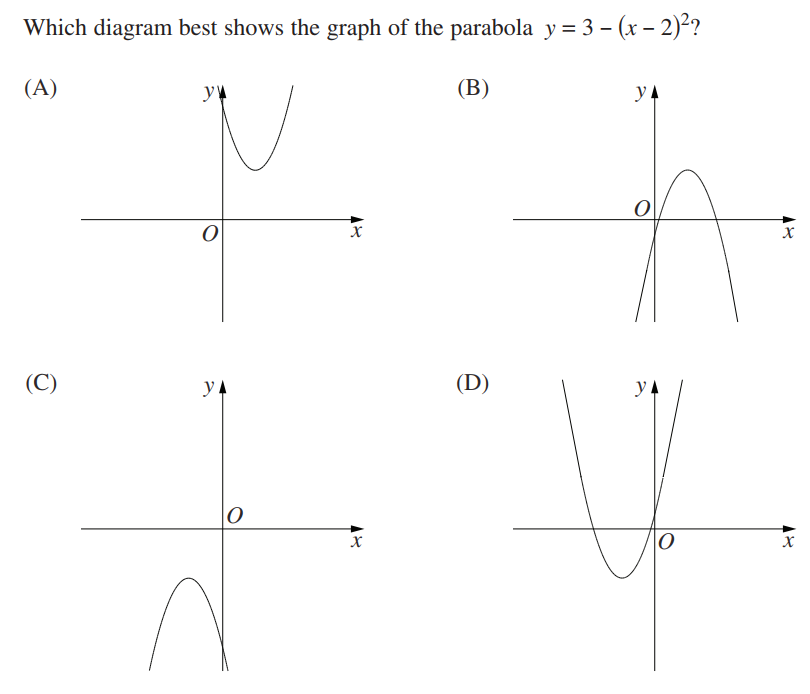###### Source: NESA Website

This HSC exam question is from the Year 11 Maths Advanced topic: ‘The quadratic polynomial and the parabola (9.1-9.5)’!

All topics from Year 11 Mathematics Advanced and/or Extension 1 are examinable in the HSC

Below is a HSC exam question from the 2016 HSC Mathematics Extension 1 Exam Paper.

2016 HSC Mathematics Extension 1 Question 10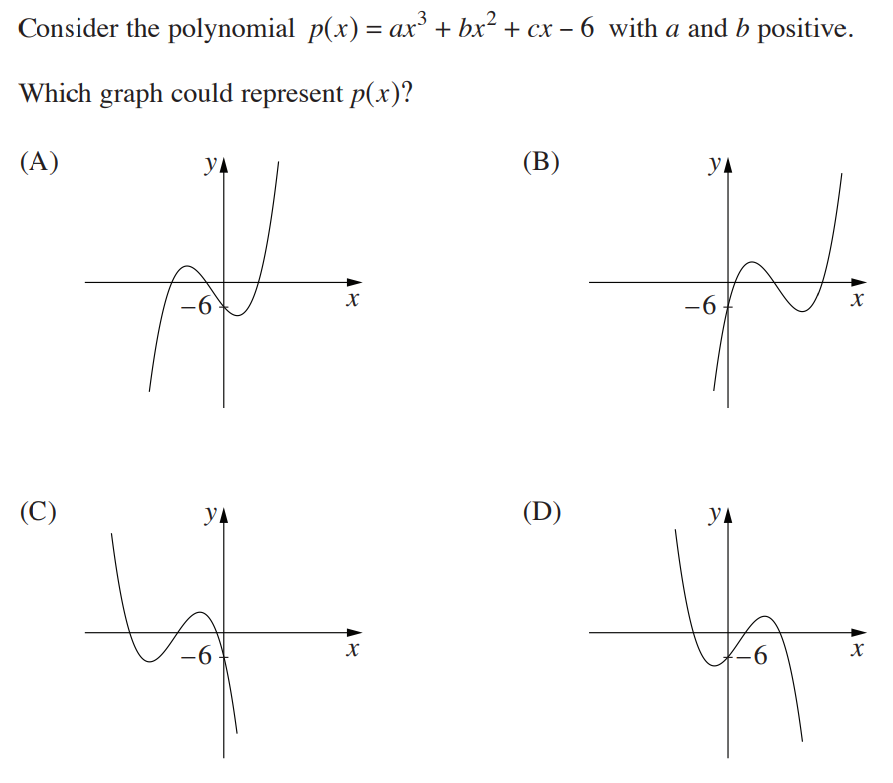###### Source: NESA Website

This HSC exam question is from the Year 11 Maths Extension 1 topic: ‘Polynomials (16.1-16.3)’!

## Scaling of Mathematics courses

Scaling is the process of converting HSC marks into scaled marks for comparison across different subjects. This conversion, or ‘scaling,’ is required as students undertake different levels of Mathematics.

A different level of scaling is applied to Mathematics courses as shown in the graph below.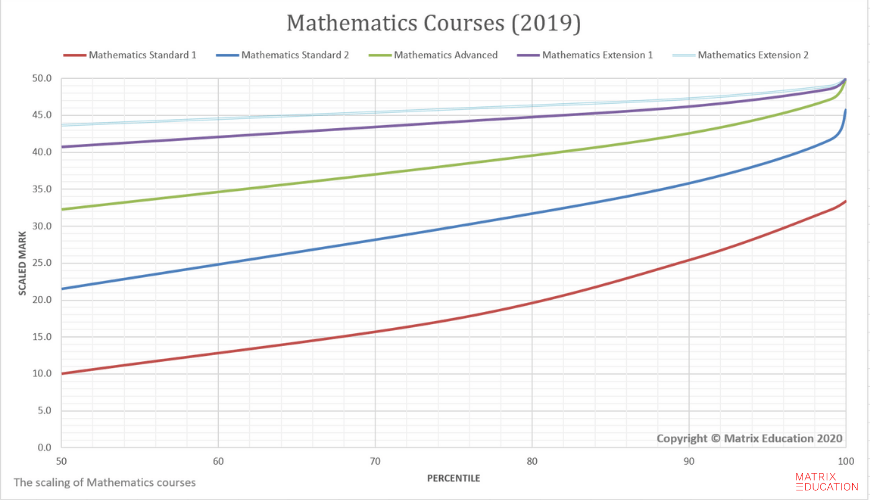Scaling of the 2019 HSC Mathematics subjects. Data from the 2019 UAC Scaling Report

As a general rule, the “harder” the unit of study, the “better” the scaling it receives.

Mathematics Extension 1 scales better than Mathematics Advanced.

Please note that, students should not choose their subjects based on scaling. Instead, scaling graphs should be used as the tool for determining your required position/rank in the state for you to obtain your desired ATAR.

For example,

• A student in 60th percentile (top 40 percent in the state) in Mathematics Extension 2 will receive a scaled mark of 45/50 which equates to 90/100, whereas a student in the 60th percentile in Mathematics Extension 1 and Advanced will receive a scaled mark of 43/50 and 34/50 which equates to 86/100 and 68/100 respectively.
• A student studying Mathematics Advanced will need to be in 97th percentile to receive the same scaled mark as the Mathematics Extension 2 student in 60th percentile.

#### Related Posts

Platform Slot Gacor CMS Checker